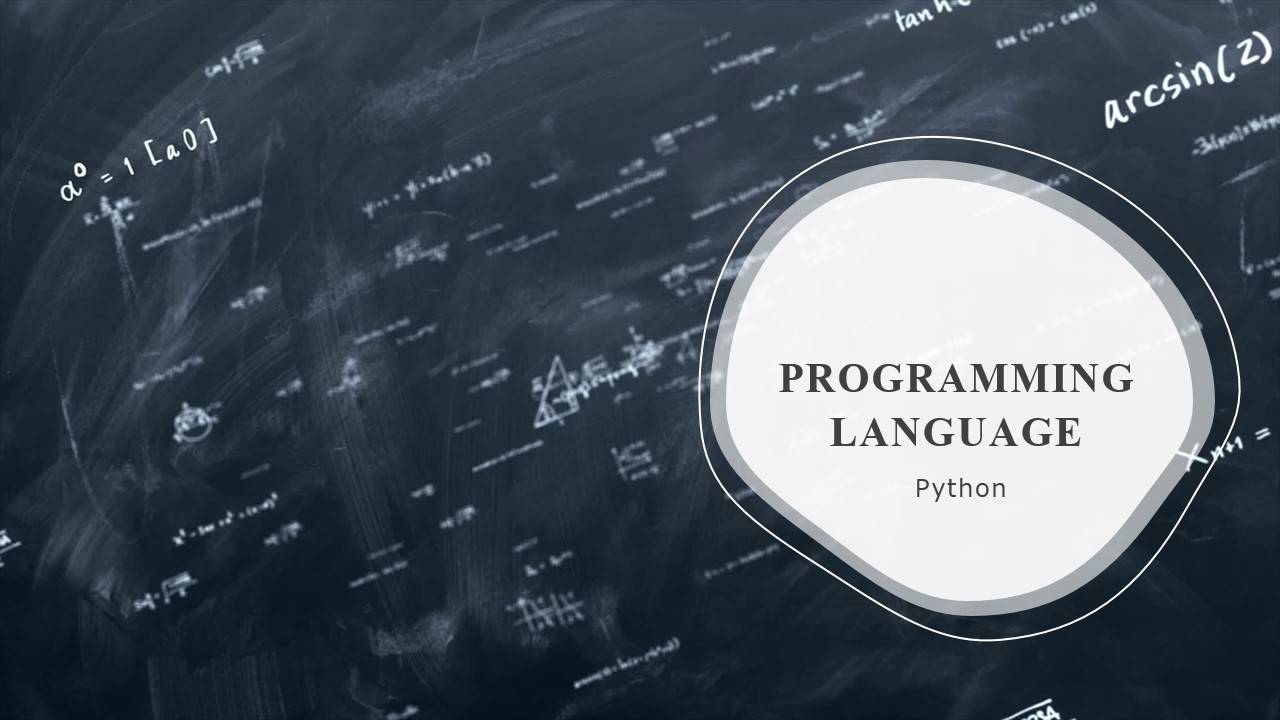/  Technology   /  What is tail recursion?## What is tail recursion?

A recursive function becomes a tail recursive function when the last thing executed by the function is the recursive call. The tail recursive functions are considered to perform better than non-tail recursive functions. This is because tail-recursion can be optimized by the compiler.

Tail Recursion

Compilers make use of stacks for the execution of recursive functions. This stack often contains crucial information like that of the variables and values for every recursive call. When a function gets called, the information after the execution gets pushed onto a stack, and after the termination, this information gets popped out of the stack. Hence, the stack size is generally more for the non-tail-recursive functions.

There’s a simple idea behind this optimization of tail-recursive functions used by the compilers. As the last statement executed is the recursive call and there’s nothing left to do in the current function, there’s no need to save the current function’s stack frame.

Can we write a non-tail recursive function as a tail-recursive to optimize it? The answer is yes.

Consider the following function which calculates the factorial of n. It’s a non-tail-recursive function. In fact, it looks like it’s in the tail call form, but since there’s multiplication that is outside of the recursive call i.e The value returned by fact(n-1) is used in fact(n), so it just can’t be optimized away.

Example:

```def factorial(n):
if n == 0:
return 1
else:
return factorial(n-1) * n```

Output:We’ll make use of one more argument and allocate the factorial value in this new argument. When n reaches 0, it returns the allocated value.

Example:

```def factorial(n, a=1):
if n == 0:
return a
else:
return```

Output: